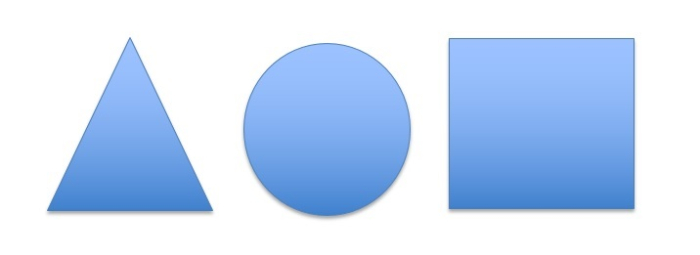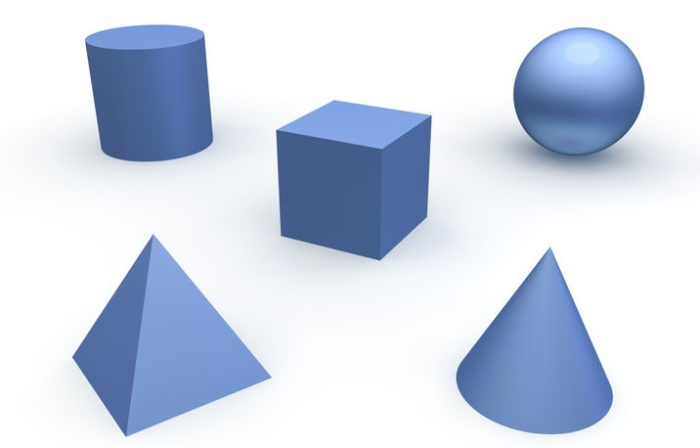# Difference Between 2D and 3D Shapes

2D shapes are flat with only length and breadth, while 3D shapes are solid objects with length, breadth, and height. In this brief article, we will take a look at the features of 2D and 3D shapes and identify how they differ from each other.

## 2D Shapes

A 2D shape has two dimensions, that is, Length and Breadth. 2D shapes are flat because they don't have any height or depth. Examples of 2D shapes include circle, rectangle, square, polygons, etc.

Since 2D shapes don't have any height, they don't have any volume either. 2D shapes have only areas. 2D shapes are drawn using X-axis and Y-axis.### Types of 2D Shapes

2D shapes are basically closed planes with no height. For example,

• A square is a 2D geometrical shape with four equal sides and four right angles.

• A rectangle has four sides and four right angles, where the opposite sides are of equal length.

• A triangle is a closed 2D plane with three sides, three angles, and three vertices.

• A circle is a two-dimensional curved shape with no corners and edges. All the points on the surface of a circle are equidistant from its center.

• An oval, just like a circle, has no corners and edges. It is a closed 2D plane with a curved face where the points on the circumference are not at equal distance from its center.

## 3D Shapes

Most of the objects that we see around us have 3D shapes, which means they have three dimensions: Length, Breadth, and Depth. Examples of 3D shapes include cubes, cylinders, spheres, cones, etc.Since 3D shapes have depth, they occupy some volume. 3D shapes are drawn using X-axis, Y-axis, and Z-axis.

### Types of 3D Shapes

3D objects come in all kinds of shapes and sizes. A sphere, for example, is a round and symmetrical 3D geometric shape with all the points on its surface at a same distance from the center. A football is a good example of a sphere.

• Cones are 3D geometrical shapes with a base and an apex point at the top. The base can either be circular or oval.

• A pyramid is another type of 3D object with a base that is a polygon and an apex. Its edges are straight, so it has flat faces. A solid triangular pyramid having four triangular faces is known as a tetrahedron. Similarly, a solid pyramid with a pentagonal base and five triangular faces that meet at an apex is known as a pentagonal pyramid.

• A cylinder is a 3D geometrical shape with two flat ends with a curved face at the middle. The two flat ends are circular.

• A torus is a 3D shape that is formed by rotating a circle in a three-dimensional space.

## Difference between 2D and 3D Shapes

The following table highlights the major differences between 2D and 3D shapes −

2D Shape3D Shape
It has two dimensions − length and breadth.It has three dimensions − length, breadth and the height.
The mathematical axes are X-axis and Y-axis.The mathematical axes are X-axis, Y-axis and Z-axis.
It is used to give a simple view of an object.It is used to give an architectural view of an object.
The edges of a two dimensional shape are clearly visible.All the edges of a three dimensional shape are not clearly visible, some are hidden.
It is easy to draw the details of a two dimensional shape.It is difficult to draw the details of a three dimensional shape.
They are easy to explain due to the clarity of the edges.Only the outer dimensions can be explained.
It is easy to draw two dimensional shapes.It is difficult to draw three dimensional shapes.
Examples include circle, rectangle, and square.Examples include cylinder, cuboid, cube, prism, etc.

## Conclusion

Most of the objects that we see around us have 3D shapes; they have height, width, and depth. In this article, we discussed why 2D objects have areas, whereas 3D objects have volumes, and also highlighted the other features of 2D and 3D objects.